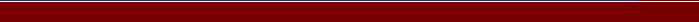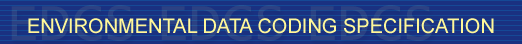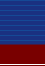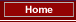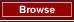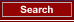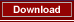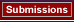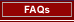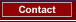## EA:  RUNWAY_END_BEARING

Status: STD 2005/06/07 1160 A two character string containing two decimal digits between "01" to "36" inclusive and defined as follows; runway end bearing. When there is a single , two parallel s, or three parallel s, the two digit whole number nearest to one-tenth of the <> (for example: a <> of 274 degrees would be represented as "27") of the centre-line of the in the direction of approach. When there are four to six parallel s, one set of two or three adjacent s shall be numbered with the two digit whole number nearest to one-tenth of the magnetic azimuth and the other set of two or three adjacent s numbered to the next nearest whole number. When these rules would give a single digit number, it shall be preceded by a zero. CONSTRAINED_STRING IR [IAN14, 5.2.2.4]